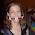## Friday, October 12, 2007

### Connecting the Dots

You have n dots on a piece of paper. How many lines do you need to connect the n dots?

The answer to this question is pretty well known, so if you want to provide the answer, please share your explanation for everyone else.

#### 7 comments:

1.Is the answer not "n-1"?

2.Well, not sure how to write this into an equation (or as an answer) but, if n was:
1, there would be 0 lines connecting
2=1
3=3
4=6
5=10
6=15
The pattern is shown in the difference between the number of lines (1,2,3,4,5)

3.n(sub x)=n(sub x-1)!

or: (I dont know if this will come out right when posted.)

n =n !
x x-1

4.no, i guess not

5.The problem as stated is too broad, it needs clarification.
If we on't consider the case of colinear points, then we could say:

it is the same as finding the number of pairs from a set of objects.
Because each pair of dots define a line.

For example:

If you have 2 points A, B in a space, then you have AB and BA, or 2*1 = 2
possibilities.
If you have 3 points A, B, C in the space, then you would have:
AB, BA, AC, CA, BC, CB or 3*2 = 6 possibilities.

You may argue that AB and BA are the same, thus giving 1 possibility
Similarly, that AB=BA, BC=CB and AC=CA, thus giving you 3 possibilities.

So, we could say that the number of pairs is 1/2 the number of
possibilities.

With n=4 points, we have 4*3/2 = 6

With n points, n(n-1)/2.

But, if we consider that the points could be colinear, then the number of lines are between 1 and n(n-1)/2.

6.Mike, I think what you were meaning is n, non-colinear points. In order to draw lines connecting n points that meet that criterion, the number of lines you would need is:

n!/(2*(n-2)!)

Basically, for each line, you're "choosing" two points, and order does not matter, so it is the general formula for "combinations" (similar to the last post, only order does matter here).

Incidentally, for our case, the above formula reduces to:

n!/(2*(n-2)!)
= (n*(n-1)*(n-2)!)/(2*(n-2!)
= n(n-1)/2

which is the same as benvitale's answer (as well as consistent with everyone else's more empirical results).

7.To say I'm impressed by these answers would be an understatement. As I said before, the answer is well known, but the explanations you gave were thorough.

I'm not really sorry the question was vague, though. To see so many possibilities explained and discussed helps me think things through, and I hope it does the same for everyone else who reads them.

Leave your answer or, if you want to post a question of your own, send me an e-mail. Look in the about section to find my e-mail address. If it's new, I'll post it soon.

Please don't leave spam or 'Awesome blog, come visit mine' messages. I'll delete them soon after.

Enter your Email and join hundreds of others who get their Question of the Day sent right to their mailbox

Preview | Powered by FeedBlitz

The Lamplight Manor Puzz 3-DAre you looking for a particular puzzle, riddle, question, etc? Or do you want to find the answer today rather than wait till tomorrow!Web statspuzzles.blogspot.com